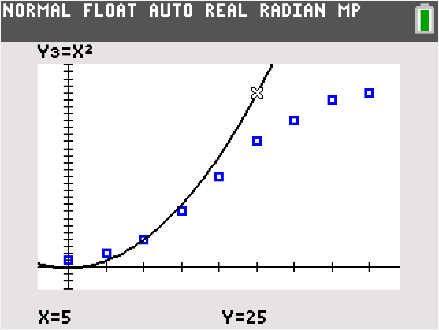# Activities

••• ##### Subject Area

• Math: Mathematical Modeling: Simulation

6-8
9-12

50 Minutes

• ##### Device
•TI-84 Plus C Silver Edition
•TI-84 Plus CE
• ##### Report an Issue#### Activity Overview

In this TI-84 family activity, students will explore a simple introduction to the idea of logistic growth through an easily acted out simulation: spread of a virus through a population.

#### Objectives

Students will be able to:

• Model a contextual situation mathematically and use the model to answer a question
• Use data from a simulation to create a scatter plot, and describe how a variable changes over time
• Determine and interpret a function modeling a quantitative variable over time